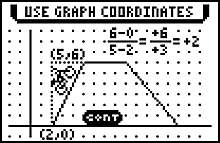# 下载操作系统、软件、应用软件、更新操作系统

## Topics in Algebra 1 (Chapters 1- 5) App for TI- 83 Plus & TI-84 Plus FamiliesThe power of an Algebra 1 tutorial on your calculator.

Topics in Algebra: Chapter 1-4 1.01 149 4
Topics in Algebra: Chapter 5 1.00 76 2

Topics in Algebra I guidebook for TI-83 Plus / TI-84 Plus (English) 浏览 2,484

## Features Summary

The power of an Algebra 1 tutorial on your calculator. Students can practice basic Algebra 1 concepts using the tutorial and practice activities to develop algebra comprehension.

Students can explore concepts such as:

• Number sense
• Linear equations
• Linear functions
• Linear inequalities

Students are also able to:

• See real problems that are modeled using linear systems
• Investigate the solution to linear systems using graphs and tables
• Review how to solve linear systems using the algebraic methods of substitution and elimination

In addition, students can download and use the worksheets below to view a step-by-step visual representation of critical concepts, then complete activities to reinforce each concept.

The Topics in Algebra I App is available for the TI-73 Explorer™, TI-83 Plus, TI-83 Plus Silver Edition, TI-84 Plus, and TI-84 Plus Silver Edition Graphing Calculators.